# Maths Quiz for Class 6, 7 and 8 (Geometry)

## Maths Quiz for Class 6, 7 and 8 with Answers (Geometry)

This maths quiz is only on “Geometry“. Class 6, 7 and 8 students from any board (CBSE, IGCSE Cambridge, ICSE, State Boards) can try this quiz out. Ten Questions are given in this Quiz. Choose the best answer from the given choices. 1 point is given for each correct answer. Also, candidates preparing for competitive exams can try this quiz out.

Correct! Wrong!

Correct! Wrong!

Correct! Wrong!

Correct! Wrong!

Correct! Wrong!

Correct! Wrong!

#### Find the angle x in the triangle shown.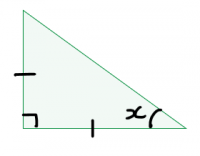Hint: You can see that this is an isosceles right triangle. Use the suitable triangle properties to solve the problem.
Correct! Wrong!

#### OA and OB are the radii of the circle. Chord AB is equal to the radius. Triangle OAB is _______________.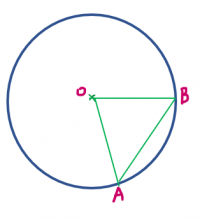Correct! Wrong!

#### The side of the triangle ABC is 6 cm (Refer to the figure). Find the value of x.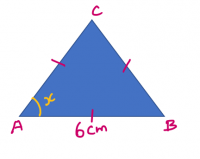Hint: ABC is an equilateral triangle. Use the suitable triangle property to solve the problem.
Correct! Wrong!

#### Which of the following is NOT true?

Correct! Wrong!

Maths Quiz 4 - for Classes 6, 7 and 8
Quiz Result: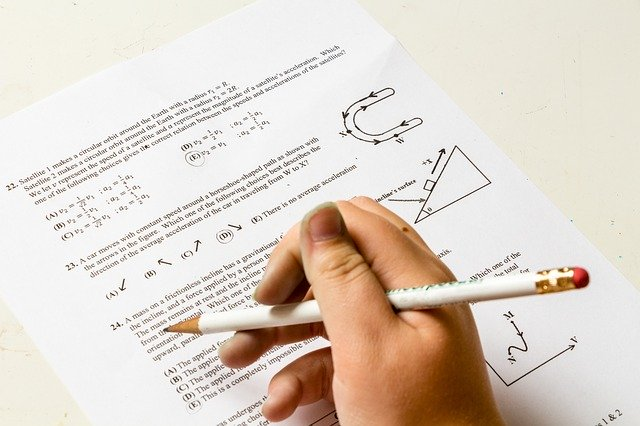Congratulations!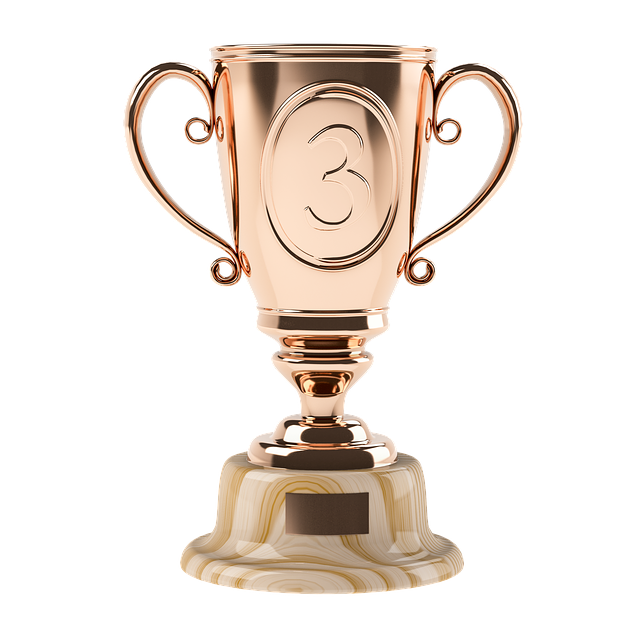Congratulations!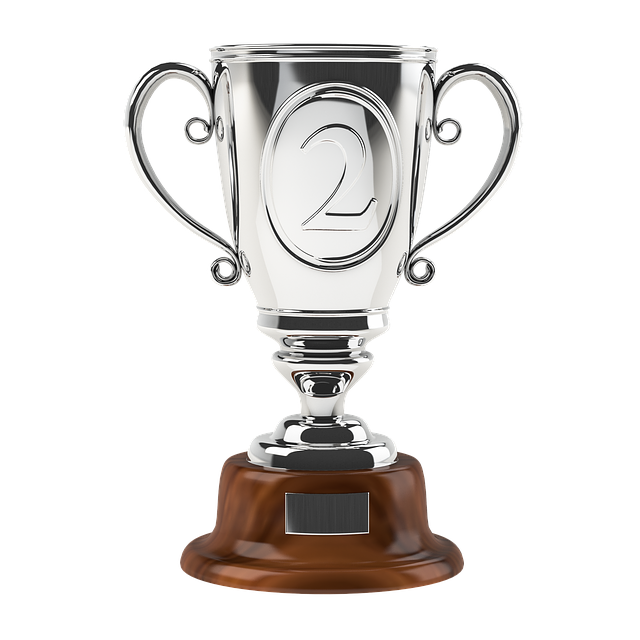Congratulations!